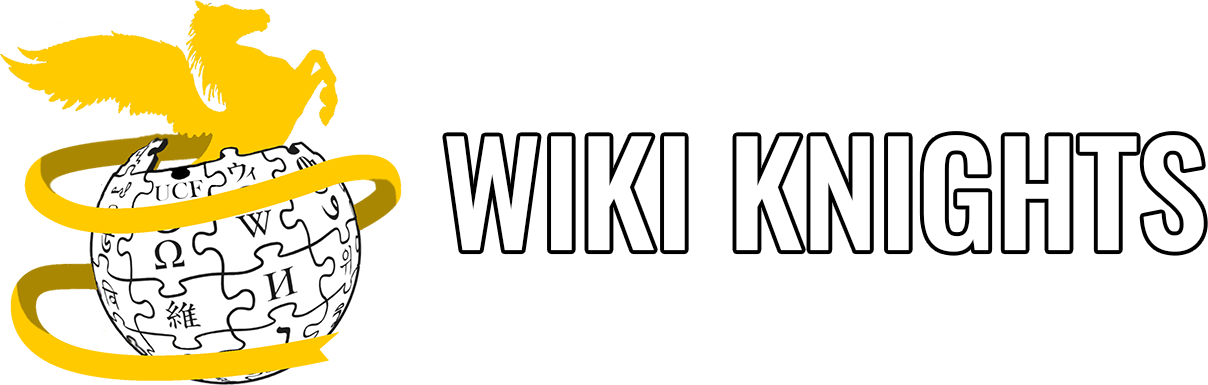# Creating and Using Functions

Originally created by Idel Martinez in Spring 2021.

## Define-ing a function.

The syntax to define a function starts with the function header, which consists of the following:

``<return_type> <function_name>([parameters]); // <---- I'm a function header!``

In here, `return_type` can be any of the types that we have learned so far:

• `int`: integer
• `float`: single-precision floating-point number
• `double`: double-precision floating-point number
• `void`: nothing, the void of emptiness

`function_name` can be anything that your heart desires, are long as it doesn’t start with a number or any of the special keywords we use in C.

`parameters` are optional (represented by the square brackets []). Because it’s optional, it means that we can define 0 or more of these parameters. How do parameters usually look like? They look like new variables, each one separated by a comma! For example, for a function called `print_error` that accepts an integer representing the error number, the parameter (and overall header) would look like this:

``````void print_error(int error);
//               ^ declaring a variable called `error` that is of type `int```````

However, a function header doesn’t do anything… Remember that the function header is like a contract we programmers define to tell C what the function should return and what it should accept, so that we don’t use it incorrectly. (You don’t want the sqrt to return nothing because it should return a double!)

To execute some code, we need the function body, which is the code that we wrap with the curly braces after a function header.

``````void print_error(int error) // <---- We got rid of the semicolon here, why?
{
// I'm in the function body!
}``````

If you took a look at the previous code block, you will see that we removed the semicolon (`;`) from the function header and added opening `{` and closing `}` curly braces to represent the function body, where we can execute the function code (whatever that may be). Remember that a semicolon represents the end of a statement, so if we add it next to a function, it means that the function has no body and, therefore, no code to execute (so why even have a function header? More on that as it develops…).

## Invoke-ing or call-ing a function.

Now that we defined the `print_error` function, which is a `void` function (meaning that it returns nothing, the void of emptiness), we can call it to run the code. Wait, what? If I write a function, doesn’t it run automatically? Simply put, no.

Say that you have the following program:

``````void print_one(void)
{
printf("I'm in print_one\n");
}

void print_two(void)
{
printf("I'm in print_two\n");
}

int main(void)
{
printf("I'm in main!\n");

return 0;
}``````

What is going to be the output?

In C, the only program that is executed (run) by default is `main` function, so we will only see

``I'm in main!``

if we run the program. To execute the code from the other two functions, we have to call them - let’s do that!

To call a function we also follow another pattern:

``<function_name>([arguments]); // <---- I'm calling a function!``

Here, `<function_name>` is the name of the function we defined earlier. In this example, we have two functions called `print_one` and `print_two`.

`arguments` are the values we pass into the function. Notice that they are not called parameters. We call parameters the variables on a function definition and arguments the variables that we pass into that function. Since both our functions accept `void`, we can call these functions with an empty parenthesis:

``````void print_one(void)
{
printf("I'm in print_one\n");
}

void print_two(void)
{
printf("I'm in print_two\n");
}

int main(void)
{
printf("I'm in main!\n");
print_one();
print_two();

return 0;
}``````

What would be the output now?

As a final exercise, determine why the following small programs are incorrect.

1. What is the value of `sum_of_numbers`?
``````int sum(int num1, int num2)
{
return num1 + num2;
}

int main(void)
{
int sum_of_numbers;

sum(1, 1);

printf("sum_of_numbers should be 2. Its value is, %d\n", sum_of_numbers);

return 0;
}``````
1. What is the output of this program? Does it run?
``````int sum(int num1, num2)
{
return num1 + num2;
}

int main(void)
{
int sum_of_numbers = sum(1, 1);

printf("sum_of_numbers should be 2. Its value is, %d\n", sum_of_numbers);

return 0;
}``````
1. Does the `result` in `main` get updated? What is its value at the end?
``````int sum(int num1, int num2, int result)
{
result = num1 + num2;

return 0;
}

int main(void)
{
int result;

sum(1, 1, result);

printf("result should be 2. Its value is: %d\n", result);
}``````